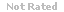Loading... Please wait...

(TCOs 1 and 2) Current issues in OSCM do not include

Price:
\$15.99

Product Description

Question 1.1. (TCOs 1 and 2) Current issues in OSCM do not include

Question 2.2. (TCO 1) Which of the following is not a characteristic that distinguishes services from goods? (Points : 4)

(TCO 5) Which of the following are used to describe the degree of error? (Points : 4)

Question 4.4. (TCO 5) The way to build in greater flexibility in your workers is to do which of the following? (Points : 4)

Question 5.5. (TCO 6) Applicants for the Baldrige Award for total quality management must submit an application of up to 50 pages that details the processes and results of their activities under seven major categories. Which of the following is one of those categories? (Points : 4)

(TCOs 3 and 7) Which of the following is considered a high-contact service operation?

Question 7.7. (TCOs 7 and 8) Which of the following is a dynamic lot-sizing technique that adds ordering and inventory carrying cost for each trial lot size and divides by the number of units in each lot size, picking the lot size with the lowest unit cost?

Question 8.8. (TCOs 4 and 8) Which of the following is a dynamic lot-sizing technique that calculates the order quantity by comparing the carrying cost and the setup (or ordering) costs for various lot sizes and then selects the lot size in which these are most nearly equal? (Points : 4)

Question 9.9. (TCO 3) Which of the following is not an improvement-driven reason to outsource? (Points : 4)

Question 10.10. (TCO 9) What transportation mode has very high initial investment costs but gives a very low cost per mile for products that are highly specialized and require no packaging? (Points : 4)

Question 1.1. (TCO 4) a company has recorded the last 5 days of daily demand on their only product. Those values are 120, 125, 124, 128, and 133. The time from when an order is placed to when it arrives at the company from its vendor is 5 days. Assuming the basic fixed-order quantity inventory model fits this situation and no safety stock is needed, which of the following is the reorder point (R)?

Question 2.2. (TCO 4) If it takes a supplier 25 days to deliver an order once it has been placed and the standard deviation of daily demand is 20, which of the following is the standard deviation of usage during lead time?

Question 3.3. (TCOs 3, 4, and 5) A company wants to forecast demand using the simple moving average. If the company uses three prior yearly sales values (i.e., year 2011 = 130, year 2012 = 110, and year 2013 =160), which of the following is the simple moving average forecast for year 2014?

Question 4.4. (TCO 5) If a firm produced a standard item with relatively stable demand, the smoothing constant alpha (reaction rate to differences) used in an exponential smoothing forecasting model would tend to be in which of the following ranges?

Question 5.5. (TCO 2) Various financial data for SunPath Manufacturing for 2012 and 2013 follow.

Question 6.6. (TCO 5) A company wants to forecast demand using the weighted moving average. If the company uses two prior yearly sales values (i.e., year 2012 = 110 and year 2013 = 130), and we want to weight year 2012 at 10% and year 2013 at 90%, which of the following is the weighted moving average forecast for year 2014?

Question 7.7. (TCO 5) If demand for product "A" were forecast at 1,000,000 units for the coming year and your factory has one machine capable of producing 75,000 units per month, how much of product "A" might you plan to acquire through outsourcing?

Question 8.8. (TCO 3) You have been called in as a consultant to set up a Kanban control system. The first thing you do is to determine the number of Kanban card sets needed. Your research shows that the expected demand during lead time for a particular component is 1,200 per hour. You estimate the safety stock should be set at 5% of the demand during lead time. The tote trays used as containers can hold two units of stock and the lead time to replenish an order is 10 hours. Which of the following is the number of Kanban card sets necessary to support this situation?

Question 9.9. (TCO 7) In a work center, machine A has a 10-minute setup time per batch and a 2 minute per unit run time. Machine B performs the identical function but has a setup time of 30 minutes and a 1-minute run time per unit. The work center makes products in batches ranging from one unit to 100 units. Assuming capacity is not a limitation on either machine, on which batches should machine B be used?

Question 10.10. (TCO 8) If annual demand is 6,125 units, annual holding cost is \$5 per unit, and setup cost per order is \$50, which of the following is the EOQ lot size?

Page 3:

1. Describe a specific example of the trade-offs between any two of the competitive dimensions
2. RspGF="font-family:'Arial';font-size:10pt;"(TCOs 3, 5, and 6) What is the difference between the concepts of design quality and conformance quality?
3. RspGF="font-family:'Arial';font-size:10pt;"(TCOs 4, 6, and 7) Distinguish between dependent and independent demand. How are these demands treated differently

Customers Who Viewed This Product Also Viewed

• \$8.99
• \$4.99
• \$8.99
• \$4.99
• \$8.99

• \$15.99